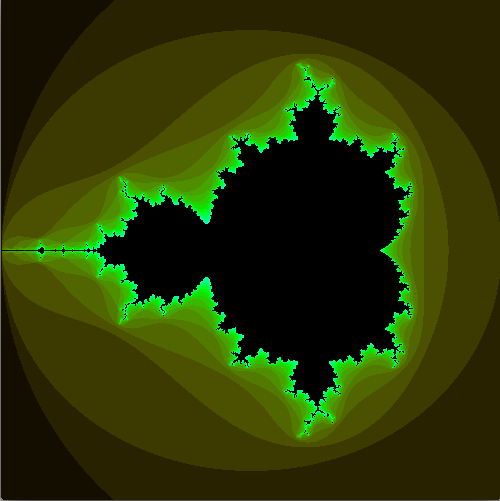# Mandelbrot Fractal

I was inspired by a My Favorite presentation from a student on the Mandelbrot fractal. I played around for a half hour at school without a ton of success, but I sorted out the sticking points on the way home, and finished it up tonight. Amazingly simple (the code may not look simple, but it’s all mine and most of it is setup. The actual fractal code is ~15 lines).Link to live openprocessing demo with source code.

Here’s the code, stripped of the color fluff.

```float fx = 0;
float fy = 0;
int count;

int resolution = 18;

void setup() {
background(0);
size(500, 500);
color c;
for (int i = 0; i < pixels.length; i++)
{
float startcx = 3.0 * (float(i % width) / width) - 2; //This provides a virtual x window from -2 to 1.
float startcy = 3.0 * (float(floor(float(i)/width))/height) - 1.5; //This provides a virtual y window of -1.5 to 1.5.
float mandelvalue = mandel(startcx,startcy);
if  (mandelvalue < 2)  c = color(0);
else  c = color(255);
pixels[i] = c;
}
updatePixels();
}

void draw()
{
}

float mandel(float startcx, float startcy)
{
float xm = 0;
float ym = 0;
count = 0;
fx = 0;
fy = 0;
while ((count < resolution) && (fx*fx+fy*fy < 4))
{
func(xm,ym,startcx,startcy);
xm = fx;
ym = fy;
count++;
}
return sqrt(fx*fx + fy*fy);
}

void func(float x, float y, float startcx, float startcy)
{
// this squares the complex number x+yi, and then adds the constant
fx = x*x - y*y + startcx;
fy = 2*x*y + startcy;
}```
This entry was posted in Full Posts and tagged , , , . Bookmark the permalink.